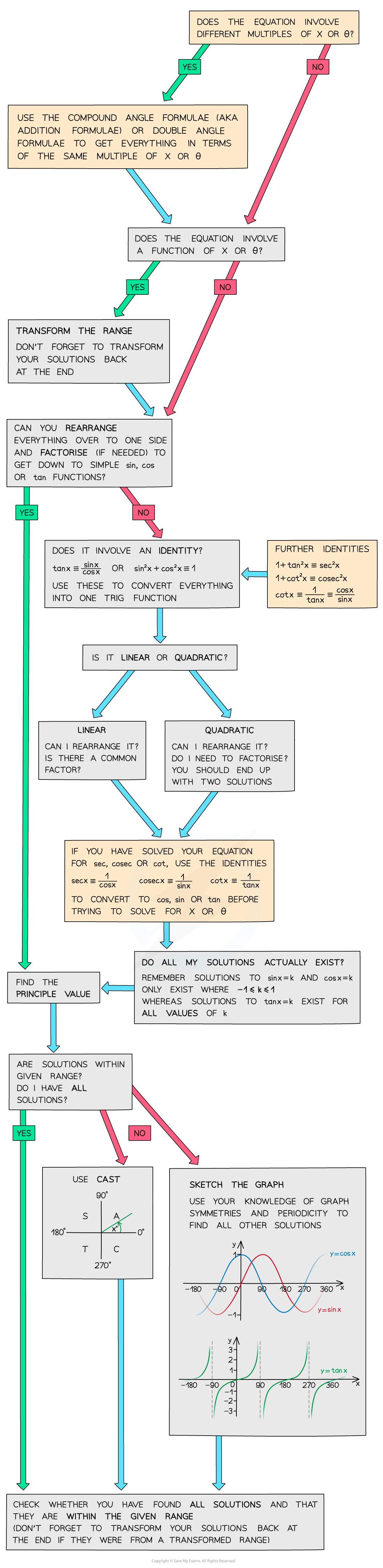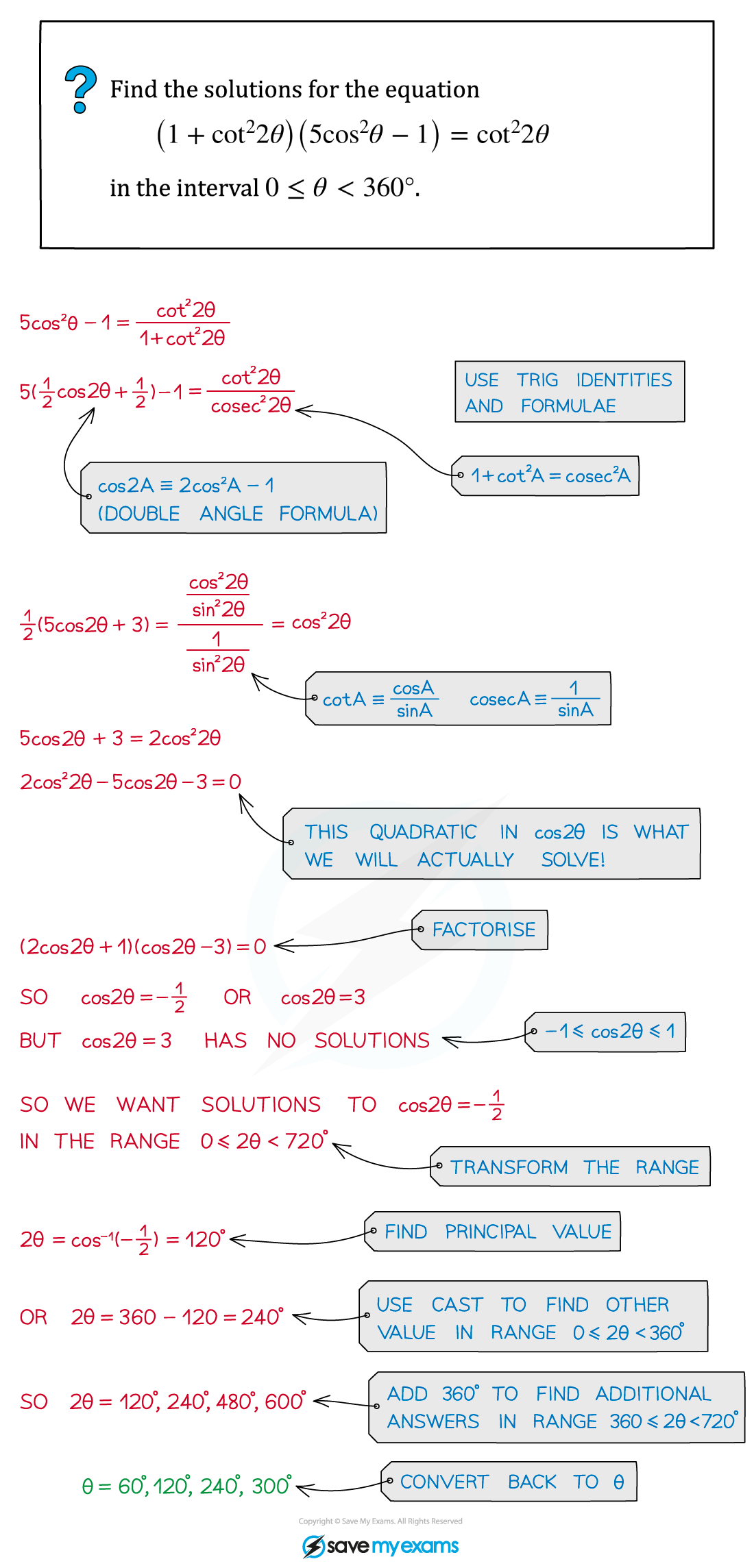# AQA A Level Maths: Pure复习笔记5.7.1 Strategy for Further Trigonometric Equations

### Strategy for Further Trigonometric Equations

#### How to approach solving harder trig equations

• You can solve harder trig equations, such as those involving reciprocal and inverse functions in a variety of different ways
• Using further trigonometric identities
• Using compound or double angle formulas
• Factorising quadratic trig equations
• Then finding all solutions using CAST or sketching graphs
• The final rearranged equation you solve will involve sin, cos or tan – don’t try to solve an equation with cosec, sec, or cot directly
• If you’re having trouble solving a trig equation, this flowchart might help:#### Exam Tip

• Try to use identities and formulas to reduce the equation into its simplest terms.
• Don’t forget to check the function range and ensure you have included all possible solutions.
• If the question involves a function of x or θ ensure you transform the range first (and ensure you transform your solutions back again at the end!).

#### Worked Example